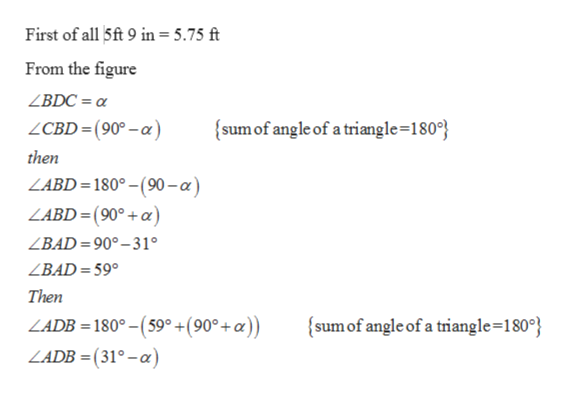# A man 5 ft 9 in tall stands on a sidewalk that slopes down at a constant angle.  A vertical street lamp directly behind him causes his shadow to be 25 ft long.  The angle of depression from the top of the man to the tip of his shadow is 31o .  Find the angle (alpha) that the sidewalk makes with the horizontal.

Question

A man 5 ft 9 in tall stands on a sidewalk that slopes down at a constant angle.  A vertical street lamp directly behind him causes his shadow to be 25 ft long.  The angle of depression from the top of the man to the tip of his shadow is 31o .  Find the angle (alpha) that the sidewalk makes with the horizontal.

check_circleExpert Solution
Step 1

Given that

A man 5 ft 9 in tall stands on a sidewalk that slopes down at a constant angle.  A vertical streetlamp directly behind him causes his shadow to be 25 ft long. The angle of depression from the top of the man to the tip of his shadow is 31o as shown

Step 2

To find out the angle alpha

Step 3help_outlineImage TranscriptioncloseFirst of all 5ft 9 in= 5.75 ft From the figure ZBDC = sum of angle of a triangle=180 ZCBD= (90°-a) then ZABD= 180°-(90-a) LABD=(90°+a) ZBAD=90°-31 ZBAD= 59° Then LADB = 180°- (59 +(90°+a)) sum of angle of a triangle=180° LADB= (31°-a) fullscreen

### Want to see the full answer?

See Solution

#### Want to see this answer and more?

Solutions are written by subject experts who are available 24/7. Questions are typically answered within 1 hour*

See Solution
*Response times may vary by subject and question
Tagged in

### Trigonometry